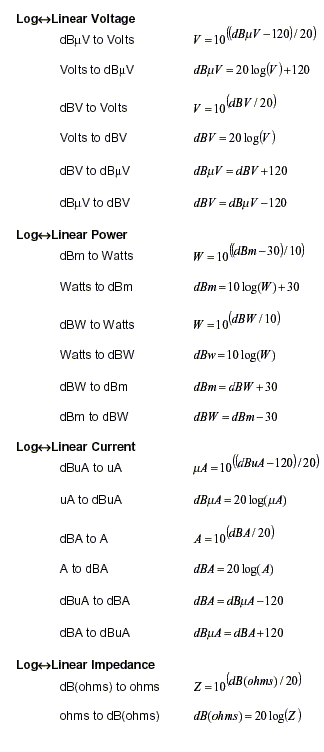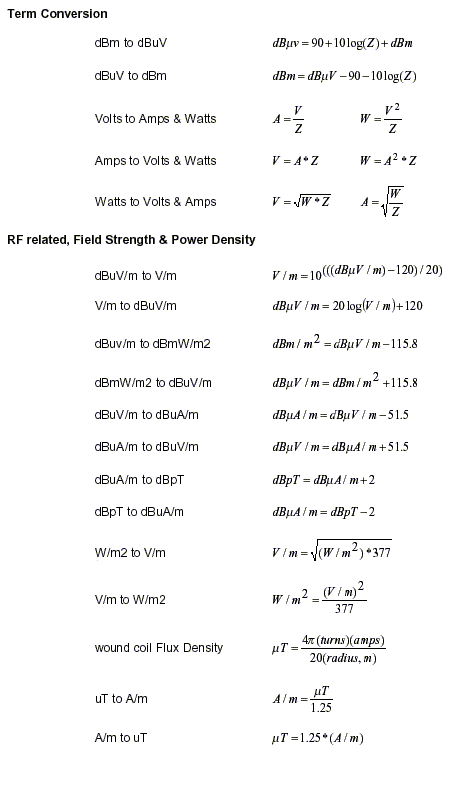Electromagnetics:  EMI/EMC Formulas, Conversions - Page 1Units dB = decibels (Log10) m = milli = 10E-3 u = micro = 10E-6 p = pico = 10E-12 dBi = decibels relative to an isotropic antenna dBw = decibels relative to one watt dBm = decibels relative to one milliwatt dBV = decibels relative to one volt dBuV = decibels relative to one microvolt dBuA = decibels relative to one microamp dBpT = decibels relative to one picoTesla V = Volts A = Amps I = Current R = Ohms (50) Resistance Z = Ohms Impedance W = Watts P = Power H = Henrys T = Teslas AF = Antenna Factor M = Meters * = Multiplication

 - Pages - 1 2